Professor Murray

Tangent and Cotangent Functions

Slide Duration:

Section 1: Trigonometric Functions
Angles

39m 5s

Intro
0:00
Degrees
0:22
Circle is 360 Degrees
0:48
Splitting a Circle
1:13
2:08
2:31
2:52
Half-Circle and Right Angle
4:00
6:24
6:52
Coterminal, Complementary, Supplementary Angles
7:23
Coterminal Angles
7:30
Complementary Angles
9:40
Supplementary Angles
10:08
Example 1: Dividing a Circle
10:38
Example 2: Converting Between Degrees and Radians
11:56
Example 3: Quadrants and Coterminal Angles
14:18
Extra Example 1: Common Angle Conversions
-1
Extra Example 2: Quadrants and Coterminal Angles
-2
Sine and Cosine Functions

43m 16s

Intro
0:00
Sine and Cosine
0:15
Unit Circle
0:22
Coordinates on Unit Circle
1:03
Right Triangles
1:52
2:25
Master Right Triangle Formula: SOHCAHTOA
2:48
Odd Functions, Even Functions
4:40
Example: Odd Function
4:56
Example: Even Function
7:30
Example 1: Sine and Cosine
10:27
Example 2: Graphing Sine and Cosine Functions
14:39
Example 3: Right Triangle
21:40
Example 4: Odd, Even, or Neither
26:01
Extra Example 1: Right Triangle
-1
Extra Example 2: Graphing Sine and Cosine Functions
-2
Sine and Cosine Values of Special Angles

33m 5s

Intro
0:00
45-45-90 Triangle and 30-60-90 Triangle
0:08
45-45-90 Triangle
0:21
30-60-90 Triangle
2:06
Mnemonic: All Students Take Calculus (ASTC)
5:21
Using the Unit Circle
5:59
New Angles
6:21
9:43
Mnemonic: All Students Take Calculus
10:13
13:11
16:48
Example 3: All Angles and Quadrants
20:21
Extra Example 1: Convert, Quadrant, Sine/Cosine
-1
Extra Example 2: All Angles and Quadrants
-2
Modified Sine Waves: Asin(Bx+C)+D and Acos(Bx+C)+D

52m 3s

Intro
0:00
Amplitude and Period of a Sine Wave
0:38
Sine Wave Graph
0:58
Amplitude: Distance from Middle to Peak
1:18
Peak: Distance from Peak to Peak
2:41
Phase Shift and Vertical Shift
4:13
Phase Shift: Distance Shifted Horizontally
4:16
Vertical Shift: Distance Shifted Vertically
6:48
Example 1: Amplitude/Period/Phase and Vertical Shift
8:04
Example 2: Amplitude/Period/Phase and Vertical Shift
17:39
Example 3: Find Sine Wave Given Attributes
25:23
Extra Example 1: Amplitude/Period/Phase and Vertical Shift
-1
Extra Example 2: Find Cosine Wave Given Attributes
-2
Tangent and Cotangent Functions

36m 4s

Intro
0:00
Tangent and Cotangent Definitions
0:21
Tangent Definition
0:25
Cotangent Definition
0:47
Master Formula: SOHCAHTOA
1:01
Mnemonic
1:16
Tangent and Cotangent Values
2:29
Remember Common Values of Sine and Cosine
2:46
90 Degrees Undefined
4:36
Slope and Menmonic: ASTC
5:47
Uses of Tangent
5:54
Example: Tangent of Angle is Slope
6:09
7:49
Example 1: Graph Tangent and Cotangent Functions
10:42
Example 2: Tangent and Cotangent of Angles
16:09
Example 3: Odd, Even, or Neither
18:56
Extra Example 1: Tangent and Cotangent of Angles
-1
Extra Example 2: Tangent and Cotangent of Angles
-2
Secant and Cosecant Functions

27m 18s

Intro
0:00
Secant and Cosecant Definitions
0:17
Secant Definition
0:18
Cosecant Definition
0:33
Example 1: Graph Secant Function
0:48
Example 2: Values of Secant and Cosecant
6:49
Example 3: Odd, Even, or Neither
12:49
Extra Example 1: Graph of Cosecant Function
-1
Extra Example 2: Values of Secant and Cosecant
-2
Inverse Trigonometric Functions

32m 58s

Intro
0:00
Arcsine Function
0:24
Restrictions between -1 and 1
0:43
Arcsine Notation
1:26
Arccosine Function
3:07
Restrictions between -1 and 1
3:36
Cosine Notation
3:53
Arctangent Function
4:30
Between -Pi/2 and Pi/2
4:44
Tangent Notation
5:02
Example 1: Domain/Range/Graph of Arcsine
5:45
Example 2: Arcsin/Arccos/Arctan Values
10:46
Example 3: Domain/Range/Graph of Arctangent
17:14
Extra Example 1: Domain/Range/Graph of Arccosine
-1
Extra Example 2: Arcsin/Arccos/Arctan Values
-2
Computations of Inverse Trigonometric Functions

31m 8s

Intro
0:00
Inverse Trigonometric Function Domains and Ranges
0:31
Arcsine
0:41
Arccosine
1:14
Arctangent
1:41
Example 1: Arcsines of Common Values
2:44
Example 2: Odd, Even, or Neither
5:57
Example 3: Arccosines of Common Values
12:24
Extra Example 1: Arctangents of Common Values
-1
Extra Example 2: Arcsin/Arccos/Arctan Values
-2
Section 2: Trigonometric Identities
Pythagorean Identity

19m 11s

Intro
0:00
Pythagorean Identity
0:17
Pythagorean Triangle
0:27
Pythagorean Identity
0:45
Example 1: Use Pythagorean Theorem to Prove Pythagorean Identity
1:14
Example 2: Find Angle Given Cosine and Quadrant
4:18
Example 3: Verify Trigonometric Identity
8:00
Extra Example 1: Use Pythagorean Identity to Prove Pythagorean Theorem
-1
Extra Example 2: Find Angle Given Cosine and Quadrant
-2
Identity Tan(squared)x+1=Sec(squared)x

23m 16s

Intro
0:00
Main Formulas
0:19
Companion to Pythagorean Identity
0:27
For Cotangents and Cosecants
0:52
How to Remember
0:58
Example 1: Prove the Identity
1:40
Example 2: Given Tan Find Sec
3:42
Example 3: Prove the Identity
7:45
Extra Example 1: Prove the Identity
-1
Extra Example 2: Given Sec Find Tan
-2

52m 52s

Intro
0:00
0:09
How to Remember
0:48
Cofunction Identities
1:31
How to Remember Graphically
1:44
Where to Use Cofunction Identities
2:52
Example 1: Derive the Formula for cos(A-B)
3:08
Example 2: Use Addition and Subtraction Formulas
16:03
Example 3: Use Addition and Subtraction Formulas to Prove Identity
25:11
Extra Example 1: Use cos(A-B) and Cofunction Identities
-1
Extra Example 2: Convert to Radians and use Formulas
-2
Double Angle Formulas

29m 5s

Intro
0:00
Main Formula
0:07
How to Remember from Addition Formula
0:18
Two Other Forms
1:35
Example 1: Find Sine and Cosine of Angle using Double Angle
3:16
Example 2: Prove Trigonometric Identity using Double Angle
9:37
Example 3: Use Addition and Subtraction Formulas
12:38
Extra Example 1: Find Sine and Cosine of Angle using Double Angle
-1
Extra Example 2: Prove Trigonometric Identity using Double Angle
-2
Half-Angle Formulas

43m 55s

Intro
0:00
Main Formulas
0:09
Confusing Part
0:34
Example 1: Find Sine and Cosine of Angle using Half-Angle
0:54
Example 2: Prove Trigonometric Identity using Half-Angle
11:51
Example 3: Prove the Half-Angle Formula for Tangents
18:39
Extra Example 1: Find Sine and Cosine of Angle using Half-Angle
-1
Extra Example 2: Prove Trigonometric Identity using Half-Angle
-2
Section 3: Applications of Trigonometry
Trigonometry in Right Angles

25m 43s

Intro
0:00
Master Formula for Right Angles
0:11
SOHCAHTOA
0:15
Only for Right Triangles
1:26
Example 1: Find All Angles in a Triangle
2:19
Example 2: Find Lengths of All Sides of Triangle
7:39
Example 3: Find All Angles in a Triangle
11:00
Extra Example 1: Find All Angles in a Triangle
-1
Extra Example 2: Find Lengths of All Sides of Triangle
-2
Law of Sines

56m 40s

Intro
0:00
Law of Sines Formula
0:18
SOHCAHTOA
0:27
Any Triangle
0:59
Graphical Representation
1:25
Solving Triangle Completely
2:37
When to Use Law of Sines
2:55
ASA, SAA, SSA, AAA
2:59
SAS, SSS for Law of Cosines
7:11
Example 1: How Many Triangles Satisfy Conditions, Solve Completely
8:44
Example 2: How Many Triangles Satisfy Conditions, Solve Completely
15:30
Example 3: How Many Triangles Satisfy Conditions, Solve Completely
28:32
Extra Example 1: How Many Triangles Satisfy Conditions, Solve Completely
-1
Extra Example 2: How Many Triangles Satisfy Conditions, Solve Completely
-2
Law of Cosines

49m 5s

Intro
0:00
Law of Cosines Formula
0:23
Graphical Representation
0:34
Relates Sides to Angles
1:00
Any Triangle
1:20
Generalization of Pythagorean Theorem
1:32
When to Use Law of Cosines
2:26
SAS, SSS
2:30
Heron's Formula
4:49
Semiperimeter S
5:11
Example 1: How Many Triangles Satisfy Conditions, Solve Completely
5:53
Example 2: How Many Triangles Satisfy Conditions, Solve Completely
15:19
Example 3: Find Area of a Triangle Given All Side Lengths
26:33
Extra Example 1: How Many Triangles Satisfy Conditions, Solve Completely
-1
Extra Example 2: Length of Third Side and Area of Triangle
-2
Finding the Area of a Triangle

27m 37s

Intro
0:00
Master Right Triangle Formula and Law of Cosines
0:19
SOHCAHTOA
0:27
Law of Cosines
1:23
Heron's Formula
2:22
Semiperimeter S
2:37
Example 1: Area of Triangle with Two Sides and One Angle
3:12
Example 2: Area of Triangle with Three Sides
6:11
Example 3: Area of Triangle with Three Sides, No Heron's Formula
8:50
Extra Example 1: Area of Triangle with Two Sides and One Angle
-1
Extra Example 2: Area of Triangle with Two Sides and One Angle
-2
Word Problems and Applications of Trigonometry

34m 25s

Intro
0:00
Formulas to Remember
0:11
SOHCAHTOA
0:15
Law of Sines
0:55
Law of Cosines
1:48
Heron's Formula
2:46
Example 1: Telephone Pole Height
4:01
Example 2: Bridge Length
7:48
Example 3: Area of Triangular Field
14:20
Extra Example 1: Kite Height
-1
Extra Example 2: Roads to a Town
-2
Vectors

46m 42s

Intro
0:00
Vector Formulas and Concepts
0:12
Vectors as Arrows
0:28
Magnitude
0:38
Direction
0:50
Drawing Vectors
1:16
Uses of Vectors: Velocity, Force
1:37
Vector Magnitude Formula
3:15
Vector Direction Formula
3:28
Vector Components
6:27
Example 1: Magnitude and Direction of Vector
8:00
Example 2: Force to a Box on a Ramp
12:25
Example 3: Plane with Wind
18:30
Extra Example 1: Components of a Vector
-1
Extra Example 2: Ship with a Current
-2
Section 4: Complex Numbers and Polar Coordinates
Polar Coordinates

1h 7m 35s

Intro
0:00
Polar Coordinates vs Rectangular/Cartesian Coordinates
0:12
Rectangular Coordinates, Cartesian Coordinates
0:23
Polar Coordinates
0:59
Converting Between Polar and Rectangular Coordinates
2:06
R
2:16
Theta
2:48
Example 1: Convert Rectangular to Polar Coordinates
6:53
Example 2: Convert Polar to Rectangular Coordinates
17:28
Example 3: Graph the Polar Equation
28:00
Extra Example 1: Convert Polar to Rectangular Coordinates
-1
Extra Example 2: Graph the Polar Equation
-2
Complex Numbers

35m 59s

Intro
0:00
Main Definition
0:07
Number i
0:23
Complex Number Form
0:33
Powers of Imaginary Number i
1:00
Repeating Pattern
1:43
Operations on Complex Numbers
3:30
3:39
Multiplying Complex Numbers
4:39
FOIL Method
5:06
Conjugation
6:29
Dividing Complex Numbers
7:34
Conjugate of Denominator
7:45
Example 1: Solve For Complex Number z
11:02
Example 2: Expand and Simplify
15:34
Example 3: Simplify the Powers of i
17:50
Extra Example 1: Simplify
-1
Extra Example 2: All Complex Numbers Satisfying Equation
-2
Polar Form of Complex Numbers

40m 43s

Intro
0:00
Polar Coordinates
0:49
Rectangular Form
0:52
Polar Form
1:25
R and Theta
1:51
Polar Form Conversion
2:27
R and Theta
2:35
Optimal Values
4:05
Euler's Formula
4:25
Multiplying Two Complex Numbers in Polar Form
6:10
Multiply r's Together and Add Exponents
6:32
Example 1: Convert Rectangular to Polar Form
7:17
Example 2: Convert Polar to Rectangular Form
13:49
Example 3: Multiply Two Complex Numbers
17:28
Extra Example 1: Convert Between Rectangular and Polar Forms
-1
Extra Example 2: Simplify Expression to Polar Form
-2
DeMoivre's Theorem

57m 37s

Intro
0:00
Introduction to DeMoivre's Theorem
0:10
n nth Roots
3:06
DeMoivre's Theorem: Finding nth Roots
3:52
Relation to Unit Circle
6:29
One nth Root for Each Value of k
7:11
Example 1: Convert to Polar Form and Use DeMoivre's Theorem
8:24
Example 2: Find Complex Eighth Roots
15:27
Example 3: Find Complex Roots
27:49
Extra Example 1: Convert to Polar Form and Use DeMoivre's Theorem
-1
Extra Example 2: Find Complex Fourth Roots
-2
Bookmark & Share Embed

## Copy & Paste this embed code into your website’s HTML

Please ensure that your website editor is in text mode when you paste the code.
(In Wordpress, the mode button is on the top right corner.)
×
• - Allow users to view the embedded video in full-size.
Since this lesson is not free, only the preview will appear on your website.

• ## Related Books1 answerLast reply by: Dr. William MurrayTue Aug 5, 2014 3:47 PMPost by Jason Wilson on July 23, 2014In example 4, TAN = SIN/COS,   is that 1/2 over   negative sqrt3 / 2 which evaluates to "- sqrt3 over 4" ?? you said one over sqrt3 that evaluates to negative sqrt3/3 please help thx1 answerLast reply by: Dr. William MurrayFri Jul 5, 2013 9:52 AMPost by Matthew Chantry on June 30, 2013The practice question for this is a secant/cosecant question, which hasn't been covered yet. This may be an error.Thanks.1 answerLast reply by: Dr. William MurrayFri Jun 21, 2013 6:16 PMPost by HAFSA Ahmad on June 12, 2013I still don't understand how to figure out the angle in radians like in this example 5pi/6 , 5pi/4 etc.2 answersLast reply by: Dr. William MurrayTue Jan 1, 2013 11:34 AMPost by Jorge Sardinas on December 31, 2012how did you turn sin[-x]/cos[-x into -sin x/cos x ; ->1 answerLast reply by: Dr. William MurrayTue Jan 1, 2013 11:25 AMPost by Ahmed Shiran on April 18, 2011This is interesting lecture ! thanks :-)

### Tangent and Cotangent Functions

Main definitions and formulas:

• The tangent function is defined by
 tanθ = sinθcosθ
for values of θ where cosθ≠ 0. (For values of θ where cosθ = 0, the tangent is undefined.)
• The cotangent function is defined by
 cotθ = cosθsinθ
for values of θ where sinθ≠ 0. (For values of θ where sinθ = 0, the cotangent is undefined.)
• Master formula for right triangles: SOHCAHTOA!
 sinθ = opposite sidehypotenuse cosθ = adjacent sidehypotenuse tanθ = opposite sideadjacent side
•  Degrees
 Cosine
 Sine
 Tangent
 Cotangent
 0
 0
 1
 0
 0
 undefined
 30
 π6
 √ 3 2
 1 2
 √ 3 3
 √ 3
 45
 π4
 √ 2 2
 √ 2 2
 1
 1
 60
 π3
 1 2
 √ 3 2
 √ 3
 √ 3 3
 90
 π2
 0
 1
 undefined
 0
• The slope of a line is the tangent of the angle that the line makes with the x-axis.
• Use these values to find sines and cosines in other quadrants. The mnemonic ASTC (All Students Take Calculus) helps you remember which ones are positive in which quadrant. (All, Sine, Tangent, Cosine)

Example 1:

Draw graphs of the tangent and cotangent functions. Label the zeroes and asymptotes of each. What are their periods?

Example 2:

A right triangle has short sides of length 3 and 4. Find the tangents and cotangents of all the angles in the triangle.

Example 3:

Determine if the tangent and cotangent functions are odd, even, or neither.

Example 4:

Find the tangent and cotangent of the following angles: (5π/6), (5π/4), (5π/3).

Example 5:

A right triangle has short sides of length 5 and 12. Find the tangents and cotangents of all the angles in the triangle.

Example 6:

Find the tangent and cotangent of the following angles: (2π/3), (7π/6), (7π/4).

### Tangent and Cotangent Functions

A triangle has short sides of length 5 and 6. Find the tangents and cotangents of all the angles in the triangle.
• First calculate the missing leg of the triangle by using the Pythagorean Theorem: a2 + b2 = c2
• 52 + 62 = x2 ⇒ 25 + 36 = x2 ⇒ 61 = x2 ⇒√{61} = x
• Draw a triangle in which the sides and angles are labeled so tangent and cotangent can be determined.
•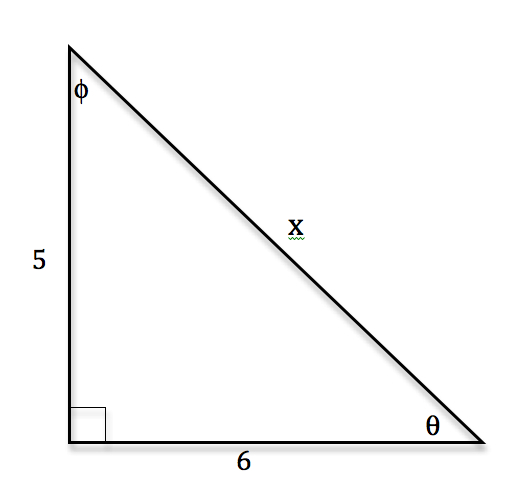tanθ = [5/6], tanϕ = [6/5], tan[(π)/2] = undefined, cotθ = [6/5], cotϕ = [5/6], cot[(π)/2] = 0
A triangle has short sides of length 9 and 11. Find the tangents and cotangents of all the angles in the triangle.
• First calculate the missing leg of the triangle by using the Pythagorean Theorem: a2 + b2 = c2
• 92 + 112 = x2 ⇒ 81 + 121 = x2 ⇒ 202 = x2 ⇒√{202} = x
• Draw a triangle in which the sides and angles are labeled so tangent and cotangent can be determined.
•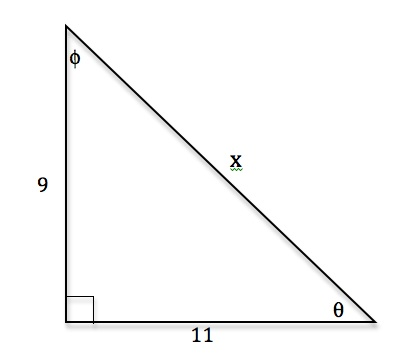tanθ = [9/11], tanϕ = [11/9], tan[(π)/2] = undefined, cotθ = [11/9], cotϕ = [9/11], cot[(π)/2] = 0
A triangle has hypotenuse of length 12 and side of length 7. Find the tangents and cotangents of all the angles in the triangle.
• First calculate the missing leg of the triangle by using the Pythagorean Theorem: a2 + b2 = c2
• x2 + 72 = 122 ⇒ x2 + 49 = 144 ⇒ 95 = x2 ⇒√{95} = x
• Draw a triangle in which the sides and angles are labeled so tangent and cotangent can be determined.
•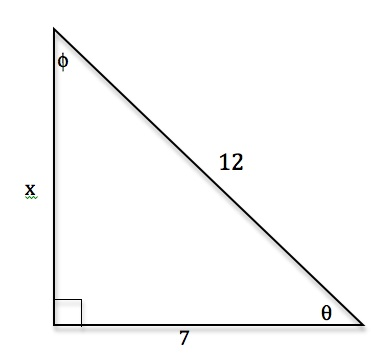tanθ = [(√{95} )/7], tanϕ = [(7√{95} )/95], tan[(π)/2] = undefined, cotϕ = [(7√{95} )/95], cotϕ = [(√{95} )/7], cot[(π)/2] = 0
A triangle has hypotenuse of length 8 and side of length 5. Find the tangents and cotangents of all the angles in the triangle.
• First calculate the missing leg of the triangle by using the Pythagorean Theorem: a2 + b2 = c2
• x2 + 52 = 82 ⇒ x2 + 25 = 64 ⇒ 39 = x2 ⇒√{39} = x
• Draw a triangle in which the sides and angles are labeled so tangent and cotangent can be determined.
•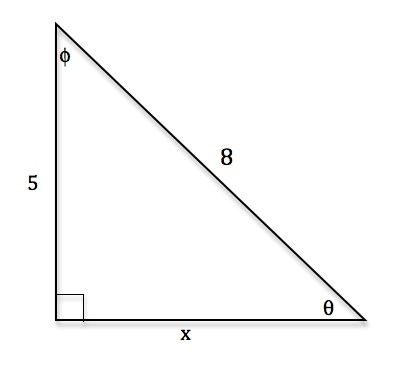tanθ = [(5√{39} )/39], tanϕ = [(√{39} )/5], tan[(π)/2] = undefined, cotθ = [(√{39} )/5], cotϕ = [(5√{39} )/39], cot[(π)/2] = 0
A triangle has hypotenuse of length 6 and side of length 4. Find the tangents and cotangents of all the angles in the triangle.
• First calculate the missing leg of the triangle by using the Pythagorean Theorem: a2 + b2 = c2
• x2 + 42 = 62 ⇒ x2 + 16 = 36 ⇒ 20 = x2 ⇒ 2√5 = x
• Draw a triangle in which the sides and angles are labeled so tangent and cotangent can be determined.
•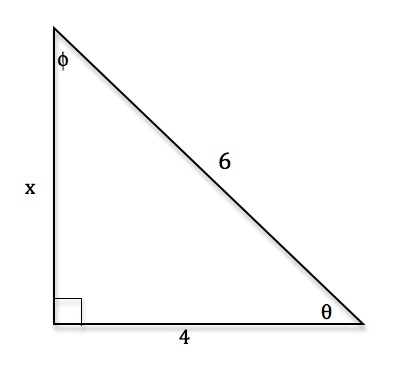tanθ = [(√5 )/2], tanϕ = [(2√5 )/5], tan[(π)/2] = undefined, cotθ = [(2√5 )/5], cotϕ = [(√5 )/2], cot[(π)/2] = 0
Find the tangent and cotangent of the following angles: [(3π)/4],[(4π)/3],[(11π)/6]
• First plot all three angles on the unit circle. Notice that [(3π)/4] uses the 45° − 45° − 90° triangle and [(4π)/3],[(11π)/6] both use the 30° − 60° − 90° triangle.
•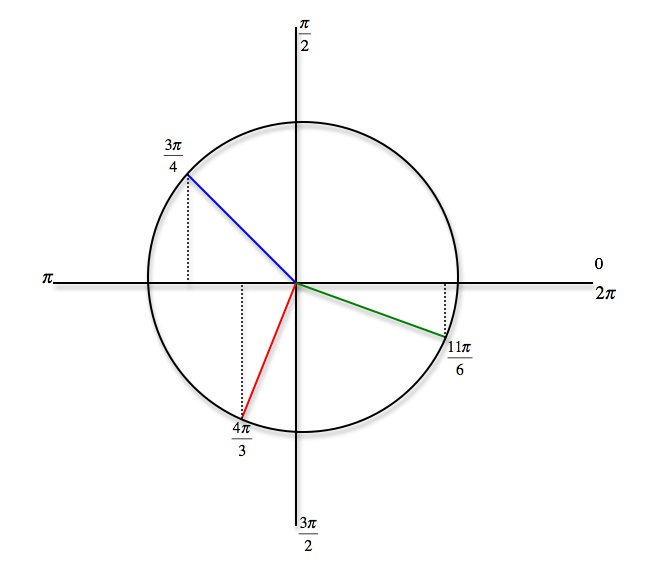• Use the mnemonic ASTC to determine which ones are positive and which are negative
 Angle sin cos tan cot [(3π)/4] [(√2 )/2] [(√2 )/2] − 1 − 1 [(4π)/3] − [(√3 )/2] − [1/2] √3 [(√3 )/3] [(11π)/6] − [1/2] [(√3 )/2] − [(√3 )/3] − √3
Sketch the graph of f(x) = tan 2x. Label the zeroes and asymptotes.
• First find two consecutive asymptotes by solving the following equations: 2x = − [(π)/2] and 2x = [(π)/2]
• So, x = − [(π)/4] and x = [(π)/4] are two asymptotes
• Use the asymptotes and sketch the tangent graph
• Zeroes are − [(π)/2], 0, [(π)/2], π, [(3π)/2], 2π, Asymptotes are [( − π)/4], [(π)/4], [(3π)/4], [(5π)/4], [(7π)/4], [(9π)/4], ...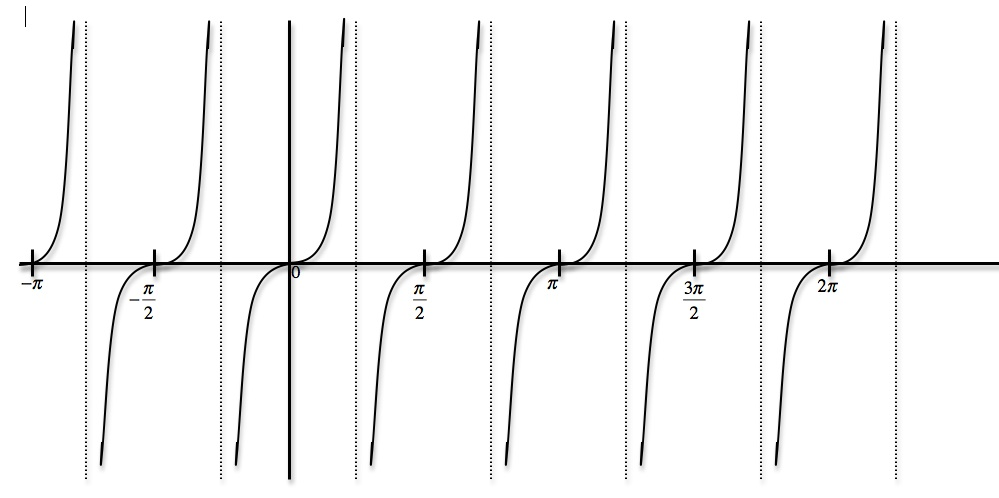Sketch the graph of f(x) = tan [x/2]. Label the zeroes and asymptotes.
• First find two consecutive asymptotes by solving the following equations: [x/2] = − [(π)/2] and [x/2] = [(π)/2]
• So, x = - π and x = π are two asymptotes
• Use the asymptotes and sketch the tangent graph
• Zeroes are 0, 2π, Asymptotes are - π, π, 3π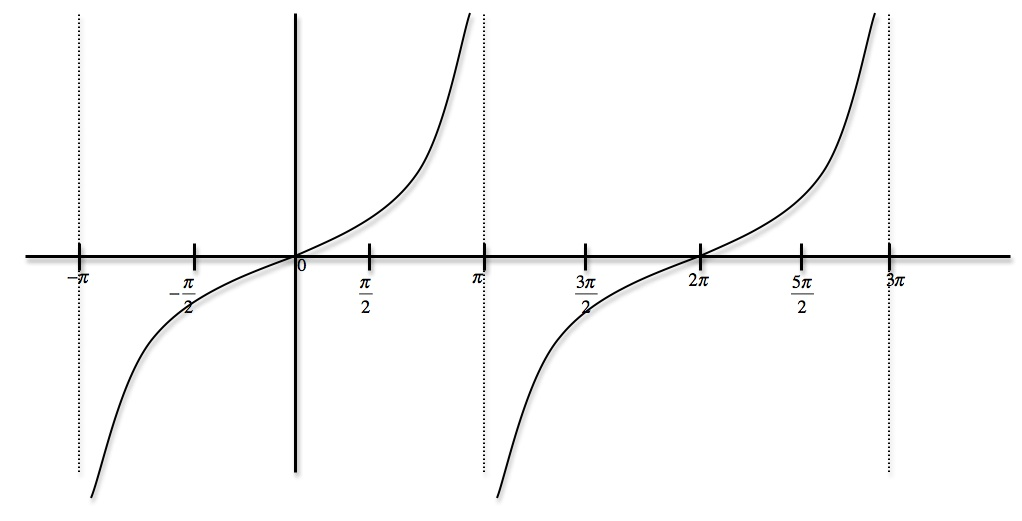Sketch the graph of f(x) = cot [x/3]. Label the zeroes and asymptotes.
• First find two consecutive asymptotes by solving the following equations: [x/3] = 0 and [x/3] = π
• So, x = 0 and x = 3π are two asymptotes
• Use the asymptotes and sketch the tangent graph
• Zeroes are − [(3π)/2], [(3π)/2], [(9π)/2], Asymptotes are 0, π, 3π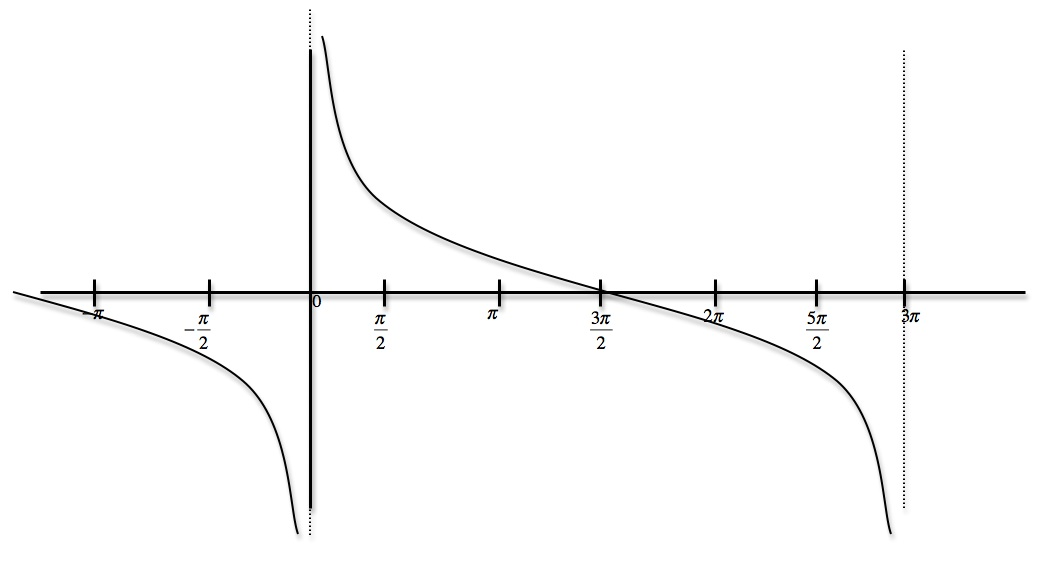Sketch the graph of f(x) = cot 4x. Label the zeroes and asymptotes.
• First find two consecutive asymptotes by solving the following equations: 4x = 0 and 4x = π
• So, x = 0 and x = [(π)/4] are two asymptotes
• Use the asymptotes and sketch the tangent graph
• Zeroes are − [(π)/8], [(π)/8], [(π)/2], Asymptotes are − [(π)/4], 0, [(π)/4], [(3π)/4]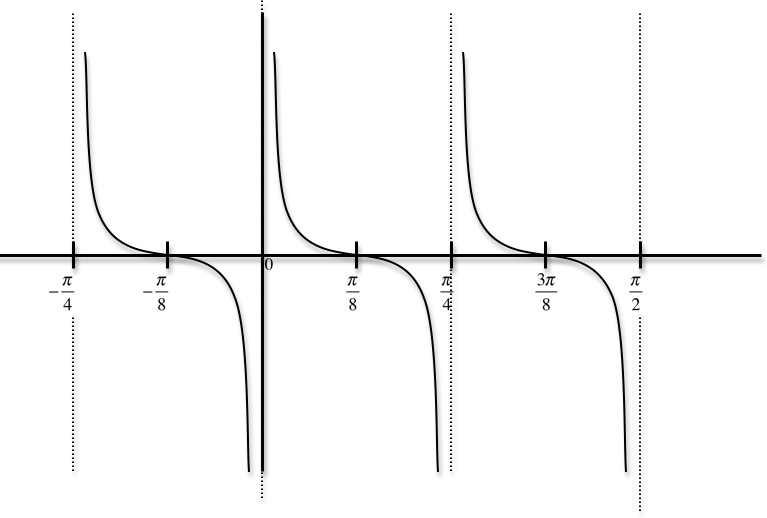*These practice questions are only helpful when you work on them offline on a piece of paper and then use the solution steps function to check your answer.

### Tangent and Cotangent Functions

Lecture Slides are screen-captured images of important points in the lecture. Students can download and print out these lecture slide images to do practice problems as well as take notes while watching the lecture.

• Intro 0:00
• Tangent and Cotangent Definitions 0:21
• Tangent Definition
• Cotangent Definition
• Master Formula: SOHCAHTOA 1:01
• Mnemonic
• Tangent and Cotangent Values 2:29
• Remember Common Values of Sine and Cosine
• 90 Degrees Undefined
• Slope and Menmonic: ASTC 5:47
• Uses of Tangent
• Example: Tangent of Angle is Slope
• Sign of Tangent in Quadrants
• Example 1: Graph Tangent and Cotangent Functions 10:42
• Example 2: Tangent and Cotangent of Angles 16:09
• Example 3: Odd, Even, or Neither 18:56
• Extra Example 1: Tangent and Cotangent of Angles
• Extra Example 2: Tangent and Cotangent of Angles

### Transcription: Tangent and Cotangent Functions

We are back with some extra examples of tan and cot functions, we are given here a right triangle with short sides of length 5 and 12.0000

Let me try to draw that and what we have to do is find the tan and cot of all the angles in the triangle.0009

Our first step there is to figure out what the hypotenuse is, hypotenuse2 is 52 + 122, which is 25 + 144 which is 169.0022

That is exactly 132, this triangle is rigged up to have a nice hypotenuse of 13 and so now let me label these angles (theta) and (phi), and we will figure out what the tan and cot of each one is.0037

The key point here, it all comes back to SOHCAHTOA.0052

We will be using SOHCAHTOA over and over again in your trigonometry so it is really worth memorizing that one.0060

If you have a hard time remembering the word, then remember the acronym Some Old Horse Caught Another Horse Taking Oats Away.0067

In particular, the tan is opposite of adjacent, tan(theta), the opposite side is 5, the adjacent side has length 12.0073

Cot(theta) is just the opposite of that, so it is 12/5.0086

Tan(phi), the opposite side for angle(phi) is 12 and the adjacent side is 5.0097

Cot(phi) is just the other way around it is 5/12.0106

Finally, the 90 degree angle a bit of special case there, let me give it in radians as pi/2.0112

Remember, tan(pi/2) is undefined and cot(pi/2) cos/sin which is 0/1 is just 0.0119

Finding cot and tan in right triangles is just a matter of remembering that mnemonics SOHCAHTOA, tan=opposite/adjacent.0136

Ok we are given here a bunch of angles and we want to find the tan and cot of each one.0000

A good first step is to graph this angles on a unit circle.0006

I got a unit circle here, I will label the common values pi/2, pi, 3pi/2, and 2pi and I want to figure out where these angles are.0019

2pi/3 is a little bit past pi/2, so we are going to find the tan and cot there.0031

7pi/6 is a little bit past pi, and 7pi/4 is in between 3pi/2 and 2pi.0038

Those are the three angles and to find their tan and cot, I will draw my triangles in and write down the sin and cos of each one.0048

For each angle I’m going to find the sin, cos, and I will use those to find the tan and cot.0061

Let us start with 2pi/3, that is over here, I will draw a triangle there, that is a 30, 60, 90 triangle.0072

I know that the sin is long one there so that must be (root 3)/2, cos of the short one is ½ except that is negative because the x coordinate is negative.0082

For tan, it is (sin/cos) that is just –root 3, cot=(cos/sin) that is -1/root 3, but that rationalizes to -root 3/3.0094

Next one is 7pi/6 so that is this angle right there, and that is a 30, 60, 90 triangle.0110

7pi/6 the (sin) is ½ but that is negative because the y coordinate is negative.0121

(Cos) the big one is root 3/2 but again that is negative because the x coordinate is negative.0128

(tan) if you divide those together, sin/cos=1/(root 3)/3 and it is positive because both of those are negative, so that 2 negative cancel.0134

(cot) is( cos/sin) that is positive root 3 because they are both negative and so the two negative is cancelled.0146

7pi/4 that is this angle down here and that is a 45, 45, 90 triangle, (sin) and (cos) are both root 2/2 for 45, 45, 90 triangle.0157

We got to figure out which one is negative and its (sin) because the (y) coordinate is negative here, we are down below the (x) axis.0177

(cos) is positive because the (x) coordinate is positive.0185

(tan) you divide those together and you get -1, and (cot) you divide (cos/sin) but you still just get -1.0188

It is probably not worth memorizing the (tan) and (cot) of those angles but it is worth knowing the common values for the 30,60,90 triangles.0202

For 30, 60, 90 it is ½, root 3/2, for the 45, 45, 90 triangles the (sin) and (cos) would be root 2/2 and root 2/2.0213

If you remember those common values then you can draw a triangle anywhere else in the unit circle and just figure out which of these values is the (sin) and which one is the (cos).0226

You can figure out which one is positive and which one is negative, to get the (tan) you just divide the (sin) and (cos) together, same for the (cot).0238

The only tricky part there is figuring the (tan) and (cot) are positive or negative, but you just look back at whether the sin and cos are positive or negative.0249

That tells you right away whether the (tan) and (cot) are positive and negative.0259

You can also remember the mnemonic all students take calculus which stands for all the values positive in the second quadrant, only the sin is positive, that is why the 2pi/3 (cot) where negative.0264

In the third quadrant, (tan) is positive which means the (cot) will be as well.0283

In the fourth quadrant, only (cos) is positive which means the (tan) will be negative.0288

A lot of different ways to remember that, choose which one works for you.0294

We will come back later with more lectures on trigonometry at www.educator.com.0298

Hi this is Will Murray for educator.com and we're talking about trigonometry.0000

We've been eluding to those in some of the earlier lectures but this is the lecture where we're going to formally define what the tangent and cotangent means and really get some practice with them.0010

The definition of tangent is very simple, it's just tangent, by definition, the tangent of an angle is sin/cos.0020

You can only talk about that when the cosine of the angle is not 0.0032

If cosine of a particular angle is 0, we just say the tangent is undefined.0039

The cotangent is just the same thing except you flip them the other way up.0043

The cotangent of an angle is cosθ/sinθ.0047

If the sinθ happens to be 0, then we say the cotangent is undefined.0053

Now, the master formula that I've already mentioned before in a previous lecture for right triangles is SOH CAH TOA.0061

You can just remember the words SOH CAH TOA or there's a little mnemonic to help you remember that if you want.0071

It's Some Old Horse Caught Another Horse Taking Oats Away.0076

If that's easier for you to remember than SOH CAH TOA, then by all means remember that.0082

The key point is that this tells you, the SOH CAH TOA formula, tells you how to interpret the sine and cosine and tangent of an angle in terms of the lengths of sides of a right triangle.0086

I've drawn a θ down here in one of the angles of a right triangle.0105

Then we talked about the side adjacent to θ and the side opposite to θ, then the hypotenuse of the right triangle.0109

The master formula that you want to remember is that the sinθ is given by the opposite side over the hypotenuse, the cosθ by the adjacent side over the hypotenuse.0128

The tanθ, which we're learning about today, is the opposite side over the adjacent side.0140

Let me run over the common values of the tangent and the cotangent function.0149

The way to remember these is to remember the common values of the sine and cosine because remember tangent is just sin/cos and cotangent is just cos/sin.0156

If you can remember the common values of sine and cosine, you can always work out the common values of tangent and cotangent.0165

I've listed the particular, the common values in the first quadrant here, in degrees, we have 0, 30, 45, 60, 90 and those correspond to radians of 0, π/6, π/4, π/3 and π/2.0173

You should really have the sines and cosines of these angles memorized to be able to reproduce them very quickly.0190

If you can, then you can immediately figure out the tangent and cotangent without really having to memorize anything extra.0199

For 0 degrees, the cosine is 1 and the sine is 0.0205

Tangent is sin/cos, that's 0/1, that gives you 0.0212

Cotangent is cos/sin, which would give you a division by 0, that's why we say it's undefined.0216

For 30 degrees, the same as π/6 radians, the cosine and sine are root 3 over 2, and 1/2.0225

Tangent, if you divide those together, you get 1 over 3.0234

If you rationalize that, that rationalizes into root 3 over 3, so that's the tangent of π/6.0244

Cotangent, if you divide them the other way, cos/sin, you just get root 3.0248

For 45, the cosine and the sine are both root 2 over 2, so the tangent and cotangent are both 1.0254

For 60 degrees or π/3, you just get the opposite you had for 30 degrees or π/6, the tangent turns out to be root 3 and the cotangent turns out to be root 3 over 3.0263

For 90 degrees or π/2, the cosine and sine are the opposite of what they were for 0 degrees, and so the tangent is undefined and the cotangent is 0.0276

The values that you get for tangent and cotangent, there's 0, 1, root 3 over 3, and root 3, and undefined.0288

Those are the common values you get for tangent and cotangent.0305

When you have one of these common angles, one of these multiples of π/6 or π/4, in degrees, that's 30 degrees or 45 degrees, it's really.0311

When you want to know the tangent or cotangent, you know it's going to be one of these common values and it's just a matter of figuring out which one.0323

It's good to remember that these common values come up over and over again because when you're working out values for any particular angle you expect it to be one of these common values.0333

There's a couple more facts that I want to talk about, uses for the tangent function.0347

One is that the slope of the line is the tangent of the angle that the line makes with the x-axis.0354

Let me try to draw that.0360

Suppose you have just a random line in a plane like this, what I'm going to do is look at the angle θ that the line makes with the x-axis.0366

Now, I'm going to move this line over.0379

I'll draw it in blue, the translated version over to the origin.0382

That's meant to be a line with the same slope that just moved over to the origin.0392

If I make a triangle like this, we still have θ in one corner of the triangle.0400

The adjacent side is equal to the amount that the line is running over, so that's the run.0411

The opposite side is equal to the rise that the line makes in that triangle.0421

If we remember SOH CAH TOA, tanθ is equal to the opposite side over the adjacent side.0432

That's the TOA part of SOH CAH TOA, which is equal to the rise over the run, which is equal to the slope of a line.0443

That tells you that for any line, the tangent of this angle θ that it makes with the x-axis is equal to the slope of that line.0455

Another thing that you want to remember about sines and cosines is that once you know the common values of tangent and cotangent.0470

If you remember which quadrants the sine and cosine are positive and negative, you can figure out which quadrants the tangent and cotangent are positive and negative.0480

Let me label the quadrants here, 1, 2, 3, and 4.0491

Let me label the positive ones.0500

Remember sine corresponds to the y value, so sine is positive in the top two quadrants, negative in the bottom two.0502

Cosine corresponds to x values, so cosine is positive in the first quadrant and in the fourth quadrant, and negative in the other two quadrants.0510

If you want to figure out when if tangent is positive, it's positive either when sine or cosine are both positive or both negative.0520

Remember, the tangent is equal to the sin/cos.0529

Tangent is going to be positive in the first quadrant, negative in the second quadrant because sine is positive, cosine is negative.0535

Tangent is positive in the third quadrant, because both sine and cosine are negative, and tangent is negative in the fourth quadrant because cosine is positive but sine is negative.0544

If you put this together, tangent is positive in the first and third quadrants, negative in the second and fourth, which is kind of the origin of this mnemonics that you can use to remember, ASTC.0557

The way you remember that is All Students Take Calculus, that stands for all the sine, cosine, tangent functions are positive in the first quadrant.0573

In the second quadrant, only sine is, in the third quadrant, only tangent is, and in the fourth quadrant, only cosine is.0585

Now, you might wonder how does cotangent fit in to all of this.0594

Cotangent, remember, is just the flip, the reciprocal of tangent, tangent was sin/cos, cotangent is cos/sin.0597

Cotangent is going to be positive whenever tangent is positive.0611

It'll be positive in the first quadrant and in the third quadrant.0614

Now we know our common values of tangent and cotangent.0622

Remember that 0, 1, square root of 3, and root 3 over 3, and we know which quadrants is going to be positive or negative, we can work out the tangent and cotangent of any common angle around the unit circle.0625

Let's try some problems now.0642

Let's start out by drawing graphs of the tangent and cotangent functions, and we're going to label the zeros and asymptotes of each and try to figure out what the periods are.0645

I'm going to start with the tangent function, and I'll do that one in blue.0662

For the tangent function, I know that the tangent of 0 is 0.0682

I'm going to put a dot at 0.0687

Let me mark my axis a bit, π/2, π, 3π/2, 2π.0690

Tangent starts out at 0.0703

The tanπ/4 we said is 1, so let me put a dot there.0710

When tangent gets close to π/2, remember that's really getting close to dividing by zero so it goes up to positive infinity there.0717

Tangent has an asymptote at π/2 and it goes up to positive infinity there, so it looks like that.0730

In the second quadrant, tangent is negative, at near π/2 it has an asymptote going down to negative infinity.0745

The tangent of π is 0 because it's sin/cos.0757

As tangent approaches 3π/2, it has another asymptote.0764

That's what the graph of tan(x) looks like.0790

That should be in blue because I'm drawing my tangent graph in blue.0799

tan(x) has zeros at 0, π, 2π, and so on.0805

It has asymptotes, basically, at the places where you're trying to divide by zero, and those are all the places where the cosine is zero.0823

Remember tangent is sin/cos.0835

Those are at π/2, 3π/2 and so on.0839

That's what the tangent function looks like.0849

Let me try to graph the cotangent function.0853

Now, the cotangent function, remember that's cos/sin, that has asymptotes and zeros exactly the opposite of where tangent did.0859

It has zeros wherever cosine is 0, which is π/2, 3π/2 and so on, because cosine is 0 at those places, 3π/2, 5π/2.0885

It has asymptotes wherever sine has 0, because we're trying to divide by 0, and that's 0, π, 2π, and so on.0907

Cotangent looks kind of just the opposite of tangent, like that.0922

That's the cotangent function in red.0965

For the next example, we're going to look at right angle triangles, and try to work out some trigonometry there.0972

We're given that a right a triangle has short sides of length 3 and 4.0977

We're trying to find the tangents and cotangents of all the angles in the triangle.0989

The first thing we need to do is figure out what the hypotenuse of this triangle is.0995

We know that the hypotenuse squared is 32+42, which is 9+16, which is 25.1000

The hypotenuse must be 5 units long.1010

Let me figure out first of all the tangents of all the angles in the triangle and we're going to use the SOH CAH TOA.1019

Remember, tangent equals opposite over adjacent.1023

The tanθ is the opposite over the adjacent which is 3/4.1030

The tanφ is the opposite side of φ which is 4/3.1040

The last angle in the triangle is 90 degrees, that's the tangent of π/2 radians, remember the tangent is undefined for π/2, so I'm just going to leave that as undefined.1050

That's because tangent is sin/cos, and cos of π/2 is 0.1069

Now, cotangent of each of these angles, cotangent is just the opposite of tangent in the sense that when tan is sin/cos, cot is cos/sin.1076

The cotθ, instead of being 3/4, will be 4/3.1089

The cotφ, instead of being 4/3, will be 3/4.1097

The cot(π/2) which is the cos/sin, that's 0/1, is just 0.1106

The secret to working out tangents and cotangents of angles in right triangles, is just to remember the SOH CAH TOA formula.1117

In particular, the TOA part, says tangent equals opposite over adjacent.1125

That quickly helps you figure out the tangents of angles and right triangles.1134

For our next example, we are asked to determine if the tangent and cotangent functions are odd, even, or neither.1138

Let's recall the definitions of odd and even functions.1144

Odd function is where f(-x)=-f(x).1149

Let me draw that a little more clearly, f(-x)=-f(x).1160

It's the kind of function that has rotational symmetry around the origin when you look at the graph.1169

An even function satisfies f(-x)=f(x) with no negative sign and that has mirror symmetry across the y-axis.1185

Those are the two definitions we want to check here.1209

Let's try that out for tangent and cotangent.1213

Tan(-x), let's see what happens.1215

Tangent by definition is sin/cos, so that's sin(-x)/cos(-x).1222

Sine remember is an odd function, sin(-x) is -sin(x), cosine is an even function so cos(-x)=cos(x).1226

You put this together, you get -sin/cos, you get -tan(x).1241

In particular, that tells you that tangent is odd.1248

We can check with the graph of tangent, it's probably worth memorizing what the graph of tangent looks like.1261

The graph of tangent look like this, it crosses the x-axis right at the origin, and then it has asymptotes at -π/2 and π/2.1271

In particular, the graph of tangent has rotational symmetry around the origin.1283

If you spun it around 180 degrees, it would look exactly the same.1290

It has that rotational symmetry around the origin, the graph of tangent definitely confirms that tangent is an odd function.1295

Let's check that out for cotangent.1304

Cot(-x), cotangent is just cos/sin, that's cos(-x)/sin(-x).1305

Just like before cos(-x)=cos(x), because cosine is an even function, sin(-x)=-sin(x), so what we get is -cos/sin, -cot(x).1318

Cotangent is odd also, it's also an odd function.1336

Again, we can check that looking at the graph.1346

Let me draw a quick graph of cot(x), probably worth remembering but not quite as essential as the tangent function.1352

If you're going to remember one of them, remember the graph of the tangent function.1360

The cotangent function looks like this, it crosses 0 at π/2, and it has asymptotes at 0 and π.1366

The cotangent function, if you draw a dot on the origin, and then rotate the thing, 180 degrees.1384

If you rotate the thing 180 degrees around the origin, the graph would look the same.1396

It has this mirror symmetry, sorry, not mirror symmetry but rotational symmetry around the origin, so the cotangent function is an odd function.1402

It does not have mirror symmetry because if you flipped it across a mirror on the y-axis, it would not look the same so it's not an even function.1415

But it does have a rotational symmetry around the origin.1425

We got another couple of examples coming up later.1429

Why don't you try them out and then we'll work them out together.1432

Okay, another example of cotangents and tangents, we're given some common values here 5π/6, 5π/4 and 5π/3.1437

Those are all angles in the unit circle.1447

We want to figure out what the tangents and the cotangents are.1451

A good way to start this is to draw the unit circle and to figure out where those angles are.1458

There's my unit circle, and let me label some easy common values, there's 0, π/2, π, 3π/2 and 2π.1478

The angles we're being asked to find, 5π/6 is just a little bit short of π, 5π/4 is in between π and 3π/2, and 5π/3 is 5/6 away around the unit circle, it's down there.1490

Those are the three angles we're being asked to find.1514

It's probably easiest if we just write down the sines and cosines of those angles, because presumably we know those pretty well.1518

Then we can figure out the tangent and the cotangent.1522

Let's make a little chart.1527

We'll find the angle, I'll find the sine and the cosine, then we'll find the tangent just by dividing those together, and the cotangent.1530

Remember the common values here.1554

The sine is the small one, that's 1/2, it's positive.1558

Cosine is the bigger one, square root of 3 over 2, and that's negative because the x value is negative there.1560

The tangent is sin/cos, so that's 1 over the square root of 3, which rationalizes to square of 3 over 3, and it's negative because the cosine was negative.1568

The cotangent is just cos/sin, so that's root 3, that's also negative.1580

Now, 5π/4, that's a 45 degree angle, South of the x-axis1588

The sine and the cosine, because it's 45 degrees, they're both root 2 over 2, but we've got to figure out whether they're positive or negative.1599

Since it's in the third quadrant there, they're both negative.1608

That means when you divide them together to get the tangent, you get a positive one.1613

When you check the cotangent, you also get a positive one.1616

Now 5π/3, that's another 30-60-90 degree angle down here.1620

The y value is the big one this time, so it's root 3 over 2, cosine is 1/2.1629

The sine is negative because we're still south of the x-axis, but the cosine is positive because we're to the right of the y-axis, meaning the x value is positive.1634

Tangent is sin/cos, that's root 3 and that's negative.1648

The cotangent is cos/sin, that's 1 over root 3, that rationalizes to root 3 over 3, that's also negative.1655

Those are our answers right there.1671

Let me just emphasize that the key to figuring this problem out is to remember the sines and cosines using the common values and those common 30-60-90 triangles and the 45-45-90 triangle.1675

As long as you remember those common values, then you can work out the sines and cosines, figure which ones are positive and which ones are negative, then divide them together to get the tangents and cotangents.1687

Of course it helps that you remember that the common values for tangent and cotangent, their always going to be 1, square root of 3, and square root of 3 over 3.1699

Then, it's just a matter of figuring out whether they're positive or whether they're negative, and which is which, for any given angle.1710

OR

### Start Learning Now

Our free lessons will get you started (Adobe Flash® required).